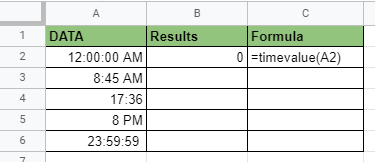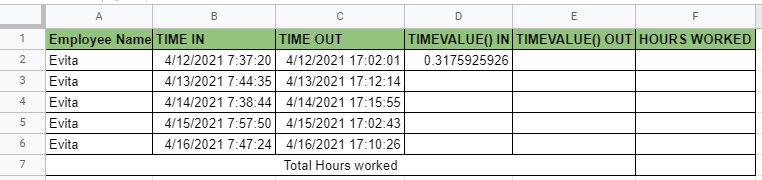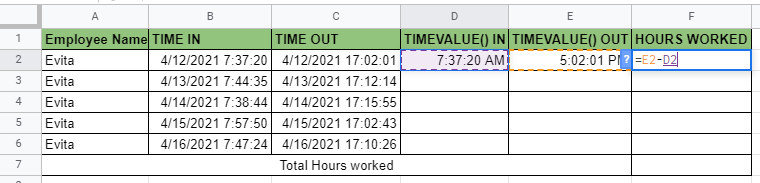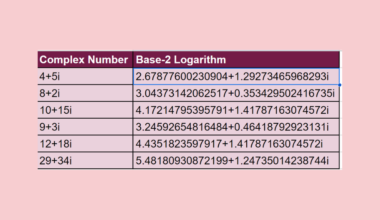# How to Use TIMEVALUE Function in Google Sheets

The TIMEVALUE function in Google Sheets is useful if you want to give a numerical value to a time in text format.

Meaning TIMEVALUE function can be used in computing for hourly wages and extracting time-related information on sources imported in text form.

The rules for using the TIMEVALUE function in Google Sheets are as follows:

• Time should be in a format that Google sheets can recognize. A good example is “7:30 PM” or “19:30”.
• Data provided will be converted to a series of decimal numbers, ranging from 0 (representing 12:00:00 AM) to 0.9999884259 (representing 11:59:59 PM).

Let’s take an example.

One of my employees, Evita, wants to know how many hours she was able to work for the week of March 12, 2021. The given data is as follows:Now we can see here the time in and time out of Evita for that specific week. However, both date and time data are combined and are in text format making it harder for us to calculate the hours.

That’s where the TIMEVALUE function in Google Sheets comes in. We can extract the time out of the data provided and put a decimal value on them. Making it much easier for us to compute for the total hours Evita worked for the week of March 12, 2021.

You can see the TIMEVALUE function in action in Column D for Time in and Column E for Time out.

Watch out for a more advanced tutorial and examples on how you can use the TIMEVALUE function in the coming weeks. Be sure to subscribe to be notified.

Perfect! Let’s begin getting to know more about our TIMEVALUE function in Google Sheets.

## The Anatomy of the TIMEVALUE Function

So the syntax (the way we write) the TIMEVALUE function is as follows:

```=TIMEVALUE(time_string)
```

Let’s dissect this thing and understand what each of these terms means:

• = the equal sign is just how we start any function in Google Sheets. It is how Google Sheets understand that we are asking it to either do computation or use a function.
• TIMEVALUE() this is our TIMEVALUE function. TIMEVALUE converts the time in the text of the “time_string” into a decimal.
• time_string is the data that provides time representation.

## A Real Example of Using TIMEVALUE Function

Take a look at the example below to see how the TIMEVALUE function is used in Google Sheets.As you can see, the TIMEVALUE function returns the fraction of a 24-hour day the time represents. You can also see on the example given different time formats that Google sheets recognize.

We can also apply different time formats available on Google sheets on our resulting data as needed, this is shown in the earlier example where we changed the hours’ format from decimal numbers to duration.

You may make a copy of the spreadsheet using the link I have attached below.

## How to Use TIMEVALUE Function in Google Sheets

1. Simply click on any cell to make it the active cell. For this guide, I will be selecting B2 where I want to show my result.1. Next, type the equal sign ‘=‘ to begin the function and then follow it with the name of the function, which is our ‘time‘ (or ‘TIME‘, not case sensitive).

1. You should find that the auto-suggest box appears with the names of the functions that all start with TIME. You may use your mouse to click on the TIMEVALUE or use your down arrow keys to highlight and hit the Enter key. Alternatively, you may completely type TIMEVALUE and hit Tab to let you use that function.1. Now the fun part! Let’s give our function the argument, which is the time_string. You may pass a constant data by typing the exact time after the parenthesis inside quotes (). Type in “12 AM”.1. Finally, just hit your Enter or Tab key. Cell B2 is now showing you the result or the return value of the TIMEVALUE function.1. Notice that in the example, we provided the exact value, constant, as the argument of our TIMEVALUE. Alternatively, this constant can simply be a cell address of your data.

1. Edit your function by changing the argument into the cell address where your time_string is located. In this case, cell A2.1. Copy the formula down to the remaining rows. Cells B2 to B6 will now show you the decimal representation of the time values in cells A2 to A6.Let’s have another practice, this time, something we can have a practical application. We will be using the earlier example we had, Evita’s total worked hours for the week of March 12, 2021.

1. We will start again by clicking on any cell to make it the active cell. For this example, I will be selecting D2 where I want to show my result.1. Next, type the equal sign ‘=‘ to begin the function and then follow it with our TIMEVALUE function.1. Now it’s time again to give our function the argument, which is the time_string. Only this time, we will be using the cell address immediately since we have the extracted data of Evita’s Time logs. In this case, we will be using B2.1. Once you hit your Enter or Tab key, the active cell should show the result or the return value of the TIMEVALUE function.1. All we have to do now is repeat the process from steps 1 to 4 in cell E2, and use C2 as our time_string argument for the TIMEVALUE function.1. Notice that in this example, even if the value we provided includes the date as the arguments of our TIMEVALUE, it still gave us a result only related to the time. We can confirm this further by changing the format of our results to Time.1. But wait, we still have to determine the hours Evita worked, right? Exactly, all we have to do now is subtract the D2 from E21. Now, this will give us a decimal result again similar to what TIMEVALUE has provided us. But don’t fret, we should be able to get the hours worked by simply changing the format again. Only this time we will be choosing Duration.1. And voila, we now have the hours worked by Evita for April 12, 2021. Now, all we have to do is copy the formula down to the remaining rows as we did in our last example. Then simply sum up all the hours worked to get the result of Evita’s all-week worked hours.1. We get 46 hours, 57 minutes, and 26 seconds total Worked hours by Evita for the week of April 12.,2021.That’s pretty much it. You can now use the TIMEVALUE function in Google Sheets together with the other numerous Google Sheets formulas to create even more powerful formulas that can make your life much easier.Our goal this year is to create lots of rich, bite-sized tutorials for Google Sheets users like you. If you liked this one, you'll love what we are working on! Readers receive ✨ early access ✨ to new content.

##### You May Also Like## How to Use TODAY Function in Google Sheets

The TODAY function in Google Sheets is useful to return the current date. We can use the TODAY…## How to Append Text to Each Cell in A Column in Google Sheets

Google Sheets has several functions in its arsenal to manipulate text which is useful when you need to…## How to Use IMLOG2 Function in Google Sheets

This guide will explain how to use the IMLOG2 function in Google Sheets. When we need to calculate…## How To Use COUPNCD Function in Google Sheets

The COUPNCD function in Google Sheets is useful when you need to calculate the next coupon date after…